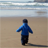USB low-full-high speed peripheralsLevel 3Level 3

# FX2 Encoder Project

I have a project that I am developing using the FX2 platform to read an I2C based encoders.  The system will host five different encoders which are read by the I2C bus and I would like to read their data buffer and place into a buffer to be read back by the computer when you do a bulk read.  This way each transaction on the USB bus yields the buffer contents of all five encoders.  I was trying to write the return of EZUSBReadI2C into EP2FIFOBUF but EP2FIFOBUF won't accept the data.

1 SolutionLevel 3Level 3

# Re: FX2 Encoder Project

Hi Yatheesh,

I was able to get the firmware to work the way I would like it to.  Thank you for the help.  To correct the return value changing from the default value to 1 instead of incrementing the default value when the encoder is turned, I added a second programming of the initial values using a EZUSB_WriteI2C(encoder, 17, (WORD)Setup_Data); .  Not reala second time.  I am not sure why this works but it does.  To get the unit to load defaults without a Vendor Request I added if(!(ENCODER_RDY))initialize_encoder(); to TD_Poll().  Now when I plug the unit in the encoders read correctly and there is no need for a vendor request unless you want to reset the default values.  Thanks for all the help!

void initialize_encoder()

{

int i, ii, iii;

BYTE Status;

BYTE xdata Setup_Data;

ii = 0;

iii =1;

//Reset the FIFO

FIFORESET = 0x80;

SYNCDELAY;

FIFORESET = 0x02;

SYNCDELAY;

FIFORESET = 0x00;

SYNCDELAY;

//Fill Encoder_Data with Setup contents

for (i=0; i < ENCODER_N; i++){

counter = default_counter;

Setup_Data = 0x00; //Register to Start

Setup_Data = 0x0B; //General Config

Setup_Data = 0x00; //Status

Setup_Data = 0x00; //Counter Byte 4

Setup_Data = 0x00; //Counter Byte 3

Setup_Data = 0x00; //Counter Byte 2

Setup_Data = 0x00; //Counter Byte 1

Setup_Data = 0x00; //Max Byte 4

Setup_Data = 0x00; //Max Byte 3

Setup_Data = 0xFF; //Max Byte 2

Setup_Data = 0xFF; //Max Byte 1

Setup_Data = 0x00; //Min Byte 4

Setup_Data = 0x00; //Min Byte 3

Setup_Data = 0x00; //Min Byte 2

Setup_Data = 0x00; //Min Byte 1

Setup_Data = 0x00; //LED A

Setup_Data = 0x00; //LED B

Setup_Data = MSB(default_counter); //Counter Byte 2

Setup_Data = LSB(default_counter); //Counter Byte 1

EZUSB_WriteI2C(encoder, 17, (WORD)Setup_Data);

EZUSB_WaitForEEPROMWrite(encoder);

EZUSB_WriteI2C(encoder, 17, (WORD)Setup_Data);

EZUSB_WaitForEEPROMWrite(encoder);

Status = Encoder_Status(encoder);

counter = default_counter;

Encoder_LED(encoder, DECREASE, 500);

}

ii = ii + 2;

iii = iii + 2;

Encoder_LED(encoder,INCREASE, 50);

}

for (i=10;i<16;i++){

EP2FIFOBUF=0xFF;

}

ENCODER_RDY = TRUE;

}

15 Replies